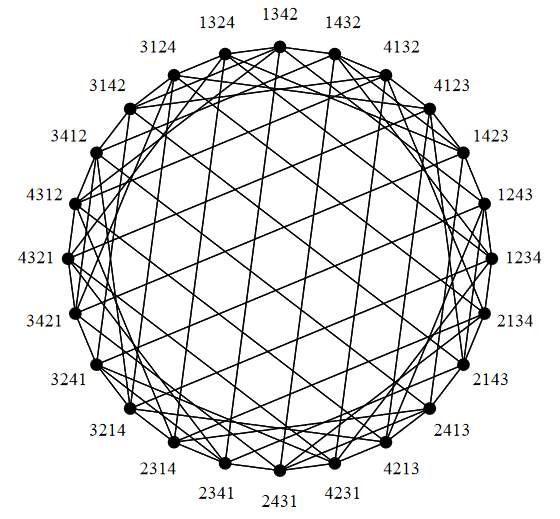Contents

group theory

# Contents

## Idea

In geometric group theory, the Cayley graph (also: Cayley quiver) of a group, $G$, equipped with a set of generators, $X$, encodes how the chosen generating elements operate (by multiplication) on the elements of the group.

## Definition

Given a group, $G$, and a set of generators, $X$ (e.g. a finitely generated group if $X$ is a finite set), which we regard as a subset of the underlying set $|G|$ of $G$, we can form a directed graph with the elements of $G$ as the vertices and with its edges labelled (some people say ‘coloured’) by the elements of $X$ with an edge joining a vertex, $g$, to the vertex $g x$ labelled by $x$

$g \overset{x}{\longrightarrow} g x$

This graph is called the Cayley graph of the group, $G$, relative to the set of generators.

The metric induced from corresponding graph distance is also called the word metric on the group $G$ with respect to its generators $X$.

## Examples

### For the symmetric group

###### Example

The symmetric group $Sym(n)$ may be generated from, in particular:

Specifically:

###### Example

(Cayley graph of Sym(3))
The following is the Cayley graph of the symmetric groups on 3 elements, $Sym(3)$, with edges corresponding to any transposition (not necessarily adjacent), hence whose graph distance is the Cayley distance:

###### Example

The Cayley graph of the symmetric group $Sym(4)$ with edges for transpositions looks as follows:graphics from Kaski 02, p. 17

###### Example

Consider one of the standard presentations of the symmetric group $S_3$, $(a,b : a^3, b^2, (a b)^2)$. Write $r = a^3$, $s = b^2$, $t = (a b)^2$.

The Cayley graph is easy to draw. There are two triangles corresponding to $1 \to a \to a^2$ and to its translate by $b$, $b \to a b \to a^2 b$, flipping the orientation of the second, and three 2-cycles, $1\to b\to 1$, $a\to a b\to a$ and $a^2\to a^2b\to a^2$.

To understand the geometric significance of this graph we compare the algebraic information in the presentation with the homotopical information in the graph (considered as a CW-complex).

Looking at the presentation it leads to a free group, $F$, on the generators, $a$ and $b$, so $F$ is free of rank 2, but the normal closure of the relations $N(R)$ is a subgroup of $F$, so it must be free as well, by the Nielsen-Schreier theorem. Its rank will be 7, given by the Schreier index formula.

Looked at geometrically, this will be the fundamental group of the Cayley graph, of the presentation. This group is free on generators corresponding to edges outside a maximal tree, and, of course, there are 7 of these.

### General

Textbooks accounts:

Review:

Discussion in homotopy type theory/univalent foundations of mathematics:

### Spectra

Discussion of Cayley graph spectra:

### Distance on Cayley graphs

On graph distances in Cayley graphs (generalizing the Cayley distance for the symmetric group):

• Farzad Farnoud (Hassanzadeh), Lili Su, Gregory J. Puleo, Olgica Milenkovic, Computing Similarity Distances Between Rankings, Discrete Applied Mathematics Volume 232, 11 December 2017, Pages 157-175 (doi:10.1016/j.dam.2017.07.038, pdf, pdf)

• Mohammad Hossein Ghaffri, Zohreh Mostaghim, Distance in Cayley graphs on permutations generated by $k m$ cycles, Transactions on Combinatorics, Vol 6 No. 3 (2017) (pdf)